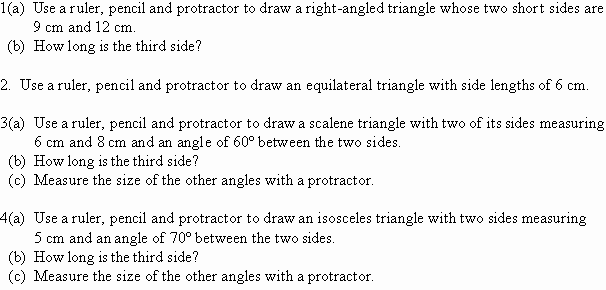Year 8 Interactive Maths - Second Edition

## Triangles

A plane is a flat or level surface in two dimensions.  Figures such as circles or squares have all of their parts lying on a plane and thus, are examples of plane figures.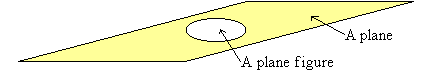A triangle is a closed plane figure bounded by three line segments.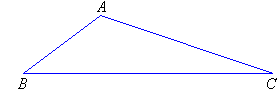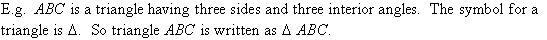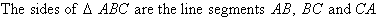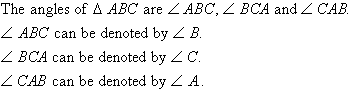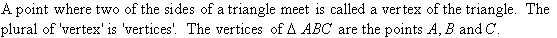## Types of Triangles

Triangles can be classified according to the length of their sides and the size of their angles.

### Classifying Triangles according to the Length of their Sides

###### Scalene Triangles

A scalene triangle has no equal sides.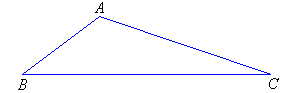###### Equilateral Triangles

An equilateral triangle has all sides equal.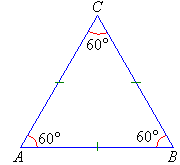All angles of an equilateral triangle are 60°.

###### Note:
• To show that the sides of an equilateral triangle have the same length, we place identical marks on the sides of the triangle.
• To show that the angles of an equilateral triangle are equal in size, we place identical curves on each angle.
###### Isosceles Triangles

An isosceles triangle has two sides equal.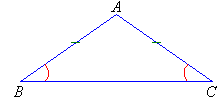The angles opposite the equal sides are equal.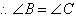###### Note:
• In the diagram, side BC has a different length to AB and AC.
• The side of the isosceles triangle that has a different length is said to be the base of the triangle.  So, BC is the base.
• Identical marks on the sides indicate that the sides are equal.
• Identical marks on the angles indicate that the angles are equal.

#### Example 8

Classify each of the following triangles according to the lengths of their sides: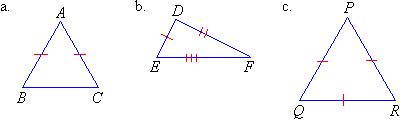##### Solution:### Classifying Triangles according to the Size of their Angles

###### Acute-angled Triangles

An acute-angled triangle has all angles less than 90º (i.e. all three angles are acute).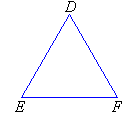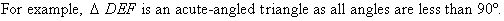###### Obtuse-angled Triangles

An obtuse-angled triangle has one angle greater than 90º.  That is, one angle is obtuse.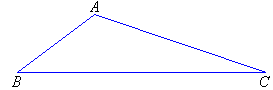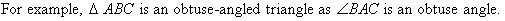###### Right-angled Triangles

A right-angled triangle has one angle equal to 90°.  That is, one angle is a right angle.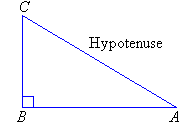###### Note:
• The side opposite the right angle is called the hypotenuse.
• The hypotenuse is the longest side of the triangle (which can be verified with a ruler).

#### Example 9

Classify each of the following triangles according to the size of their angles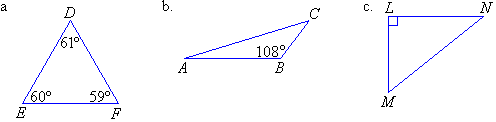##### Solution: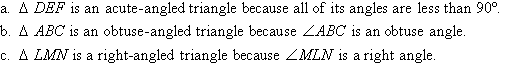### Activity 9.1